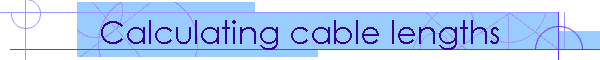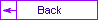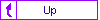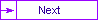+How do you calculate the length of the balloon cables? Below are the calculations that I used.

 Calculating Cable Lengths for Hot Air Balloons Input Mouth Diameter 15 Basket Attachment Diameter 1 2/8 Angle of mouth 46.08 Output Formula Theoretical Cable Length 10 3/8 Mouth Radius/SIN(Angle of Mouth) Amount off bottom 7/8 Attachment Radius/SIN(Angle of Mouth) Actual Cable Length 9 4/8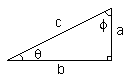sin(theta) = a / c csc(theta) = 1 / sin(theta) = c / a cos(theta) = b / c sec(theta) = 1 / cos(theta) = c / b tan(theta) = sin(theta) / cos(theta) = a / b cot(theta) = 1/ tan(theta) = b / a

I used the upper left equation here and solved for C to get my formula for the theoretical cable length. One thing to make sure of when you do this, make sure you don't mix degrees and radians! Most SIN functions assume radians for input.

As for the angle of the mouth, Bob Nungster's program put out the value that I am using. Bob LeDoux uses 50 degrees in one of his BBJ articles. In a later article he corrects it to a different value, but I do not know what that value is. I emailed him and he recommended using 40 degrees.

My 65k 1987 TBW balloon has a cable length of 9 feet. On my balloon the actual length of the cables vary by as much as 5 inches. At first I was not sure why. Later I asked Sid from the Balloon Works, and he reminded me that since the cables start at one point and fan out, the two outer cables have to be longer than the two inner cables.